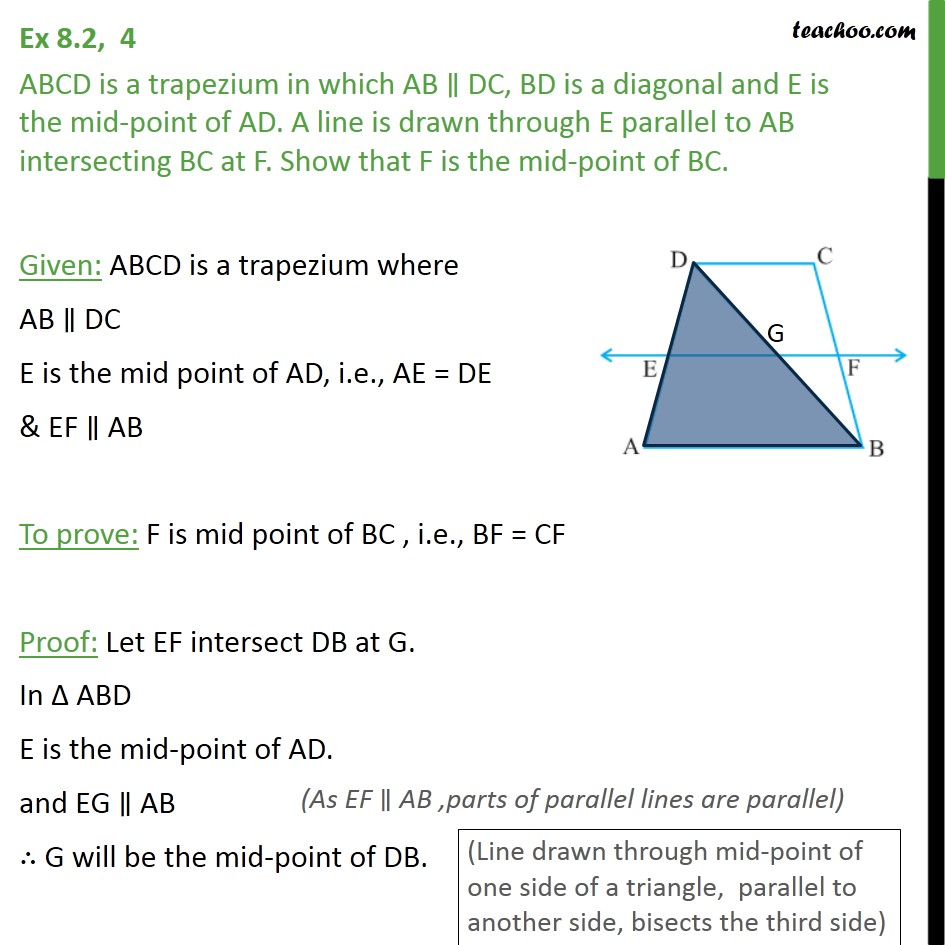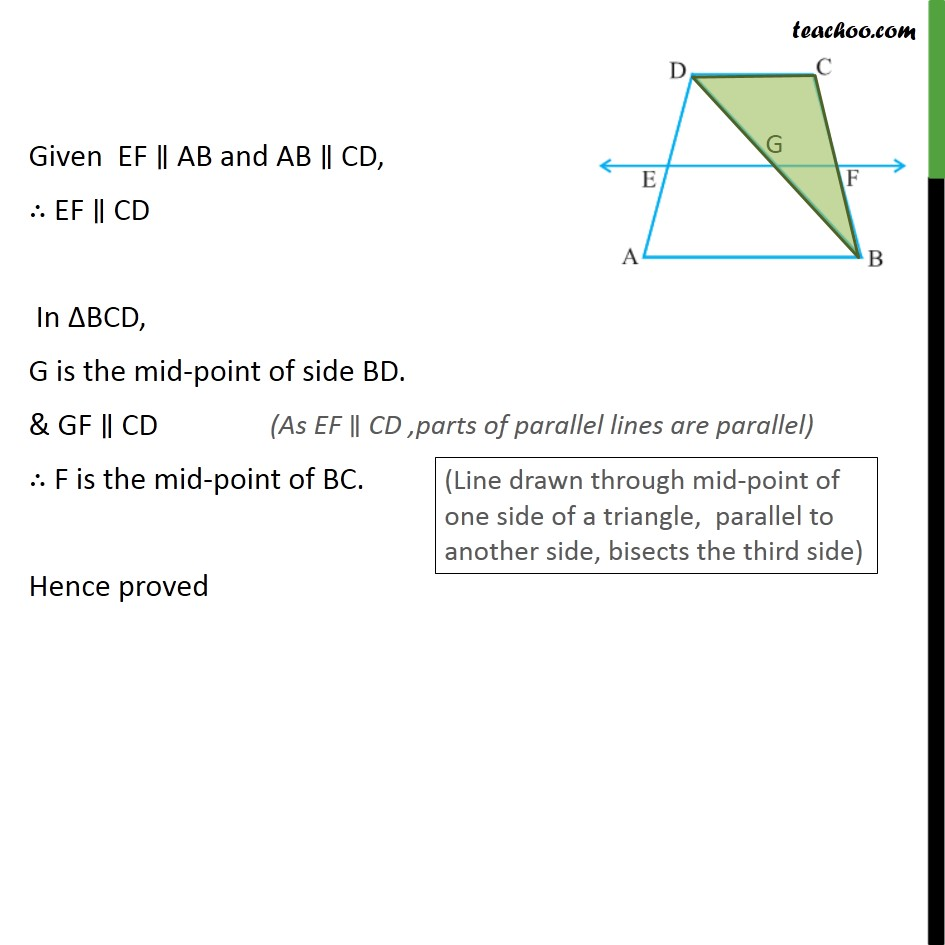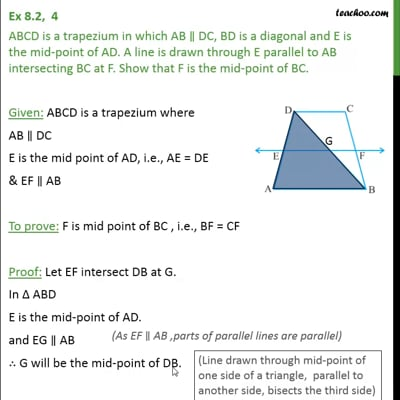Class 9
Important Questions for Exam - Class 9This video is only available for Teachoo black users

### Transcript

Ex 8.2, 4 ABCD is a trapezium in which AB ∥ DC, BD is a diagonal and E is the mid-point of AD. A line is drawn through E parallel to AB intersecting BC at F. Show that F is the mid-point of BC. Given: ABCD is a trapezium where AB ∥ DC E is the mid point of AD, i.e., AE = DE & EF ∥ AB To prove: F is mid point of BC , i.e., BF = CF Proof: Let EF intersect DB at G. In ∆ ABD E is the mid-point of AD. and EG ∥ AB ∴ G will be the mid-point of DB. Given EF ∥ AB and AB ∥ CD, ∴ EF ∥ CD In ΔBCD, G is the mid-point of side BD. & GF ∥ CD ∴ F is the mid-point of BC. Hence proved